# Summery

You Can find the results from this research on my `Github` here:

## Yara Rule for Detection

AveMaria(WareZone)RAT

## Python Configuration Extractors

There are two Configuration Extractors(the explanation mentioned in the blog post)

If standerd RC4

If NonStanderd RC4

# OverView

This will not be a detailed analysis of the sample as I did in a previous blog post you can find it here “AveMariaRAT Detailed Analysis”. Instead, I will cover how to Detect it using a Yara rule and testing it against a large number of samples, and also writing a Configuration extractor for it.

I am using two samples to test my yara when writing the rule and then I will extend the testing against a bigger number of samples once finished.

# where to start?!

This a good question and today there is one that is like to be asked which is chatGPT so why not to ask him?!!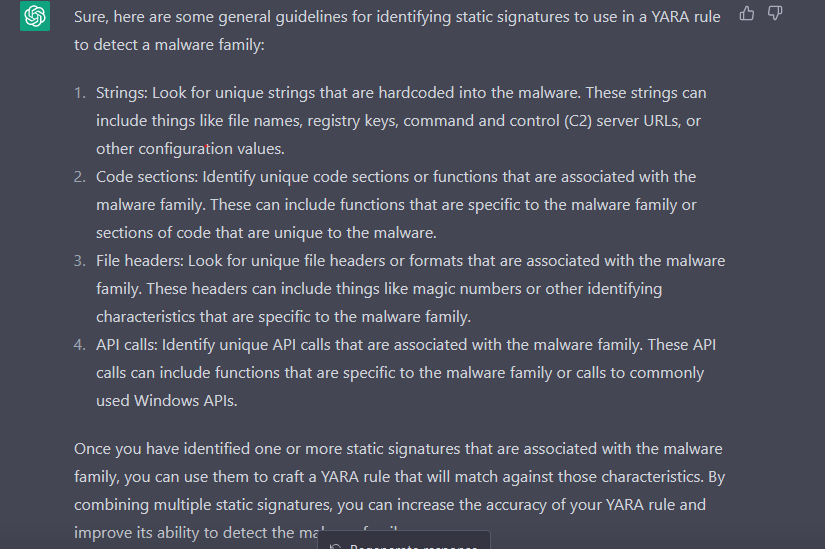As we are looking for PE files we will specify the “MZ” header to be at the beginning of the file.

``````  \$mz = {4D 5A}     //MZ header
``````

And I added also a file size check to look for files smaller than 300k

``````filesize < 300KB
``````

# Strings

It’s a good place to start looking for Individuals.

I found some strings that may look promising.

``````cmd.exe /C ping 1.2.3.4 -n 4 -w 1000 > Nul & cmd.exe /C
cmd.exe /C ping 1.2.3.4 -n 2 -w 1000 > Nul & Del /f /q
``````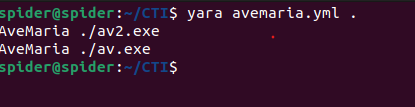Until now the yara detects both of the samples so let us continue.

# Byte Sequence

AveMaria is known to have PE files encrypted in the resources section and in some place in the file it will be read and decrypted.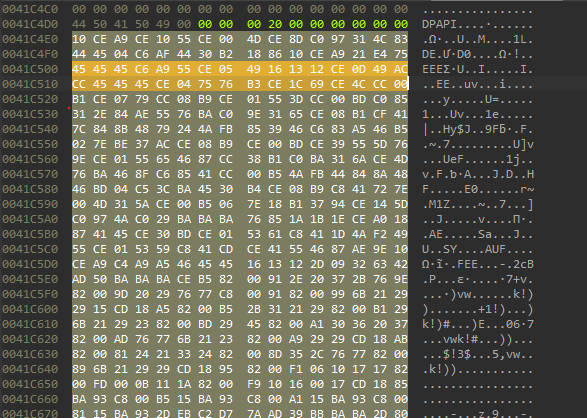# Code Sections

In this area we have different options The first one is performing manual analysis for functions and looking for the functions that will luckily be the same, But as I said I won’t do a detailed analysis, So I will go with the second option which is using similarity between the two samples that we have using a binary diffing tool like `bindiff` plugin.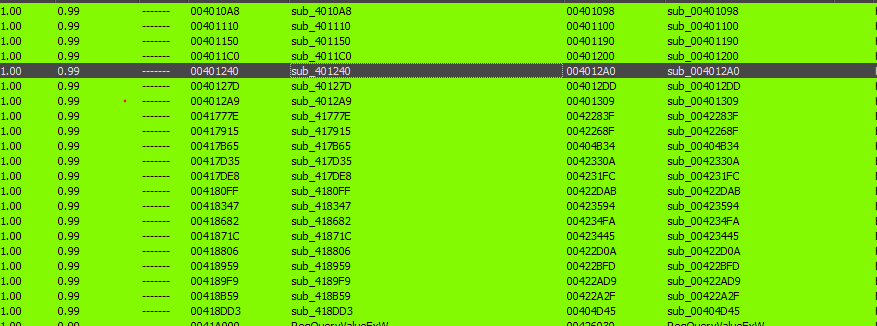A good place to look at when looking for persistent code sections is the decryption algorithms.

look At this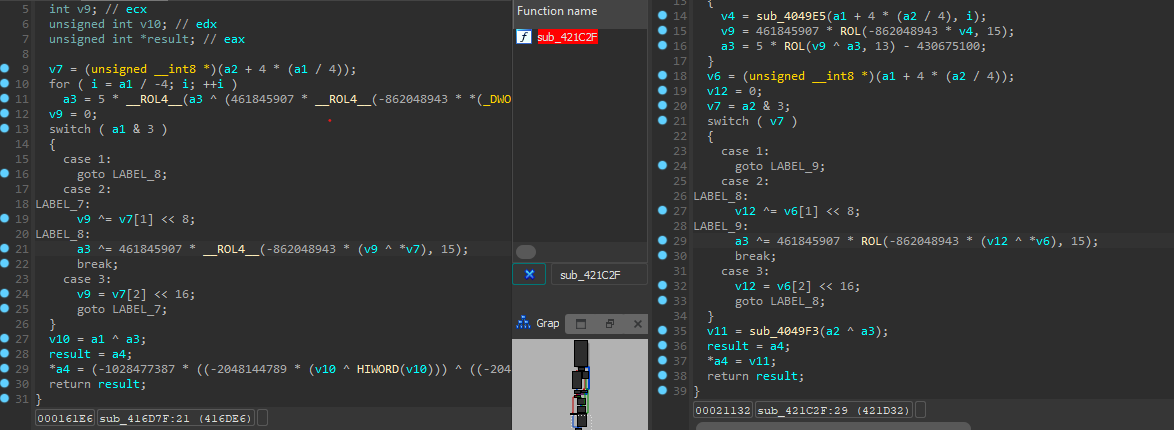You can notice that the functions are identical In the operation but not in the byte code sequence, So I decided to use the integer values “Keys” and add them to my rule.

# Final 1 Before Testing

So until now this is our rule

``````rule AveMaria : RAT
{
meta:
description = "Detection Rule for AveMaria(warzone) RAT"
email = "amr.ashraf.re@outlook.com"
author = "Amr Ashraf"

strings:
\$mz = {4D 5A}     // MZ header

\$string1 = "cmd.exe /C ping 1.2.3.4 -n 4 -w 1000 > Nul & cmd.exe /C"
\$string2 = "cmd.exe /C ping 1.2.3.4 -n 2 -w 1000 > Nul & Del /f /q"

\$K_1 = {35 AE B2 C2}
\$K_2 = {6B CA EB 85}

\$rcrs_seq = {45 45 45 C6 A9 55 CE 05 49 16 13 12 CE 0D 49 AC CC 45 45 45 CE 04 75 76 B3 CE 1C 69 CE 4C CC 00}

condition:
\$mz at 0 and
2 of (\$string*) or
\$rcrs_seq and
\$K_1 and \$K_2 and
filesize < 300KB
}
``````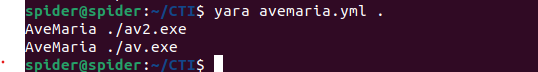And it actually caught both of the samples that I am working on, But definitely, We can’t depend on that to say that it’s working because we used them in the process of writing, so we need to get more samples and test our rule against them.

# Retrive Samples

There are two free services that I use to retrieve samples via API, they are `Triage` and `MalwareBazaar`

You can use this code to download Samples from triage just pass the family name and how many samples you need.

``````import requests
import json
import argparse
import os

# Set up the command line argument parser
parser = argparse.ArgumentParser(description="Fetch samples from the Triage API")
parser.add_argument("query_string", help="The search query string to use")
parser.add_argument("num_ids", type=int, help="The number of sample IDs to fetch")

# Parse the command line arguments
args = parser.parse_args()

# Replace <YOUR_ACCESS_KEY> with your access key
access_key =  "<YOUR_ACCESS_KEY>"

# Set the API endpoint URL for searching samples
search_url = f"https://tria.ge/api/v0/search?query=family:{args.query_string}"

# Set the request headers to include the access key

# Send the GET request to the API endpoint for searching samples

# Check if the request was successful (status code 200)
if response.status_code == 200:
# Parse the JSON response and extract the value of the "id" key
id_list = []
for data in resp["data"][:args.num_ids]:
id_list.append(data["id"])
print(f"Fetched sample ID: {data['id']}")

# Set the API endpoint URL for retrieving samples by ID
sample_url_prefix = "https://tria.ge/api/v0/samples/"
for sample_id in id_list:
sample_url = sample_url_prefix + sample_id + "/sample"

# Send the GET request to the API endpoint for retrieving the sample

# Check if the request was successful (status code 200)
if response.status_code == 200:
# Save the sample data to a binary file named after the sample ID
filename = f"{sample_id}.bin"
with open(filename, "wb") as f:
f.write(response.content)
print(f"Saved sample ID {sample_id} to {filename}")
else:
print(f"Error retrieving sample ID {sample_id}: {response.status_code}")
else:
print(f"Error searching for samples: {response.status_code}")
``````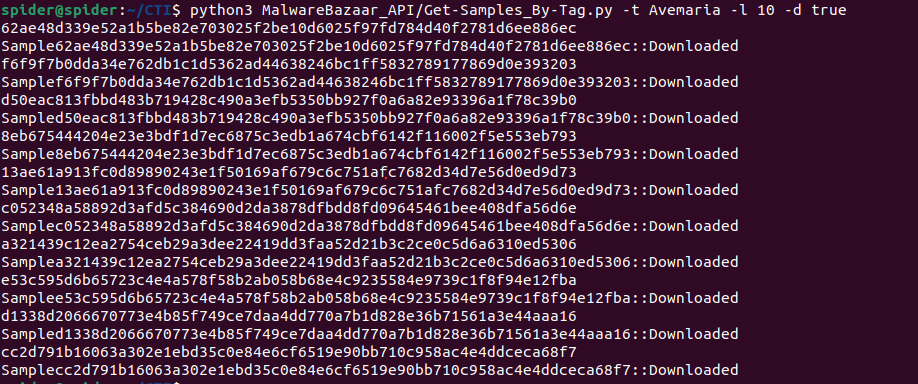Now we need to use an unpacking service like “unpac.me” to speed up the process of unpacking.

# Yara Testing

After unpacked more samples to test my rule against. It catched them all.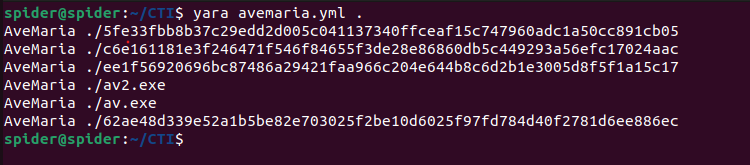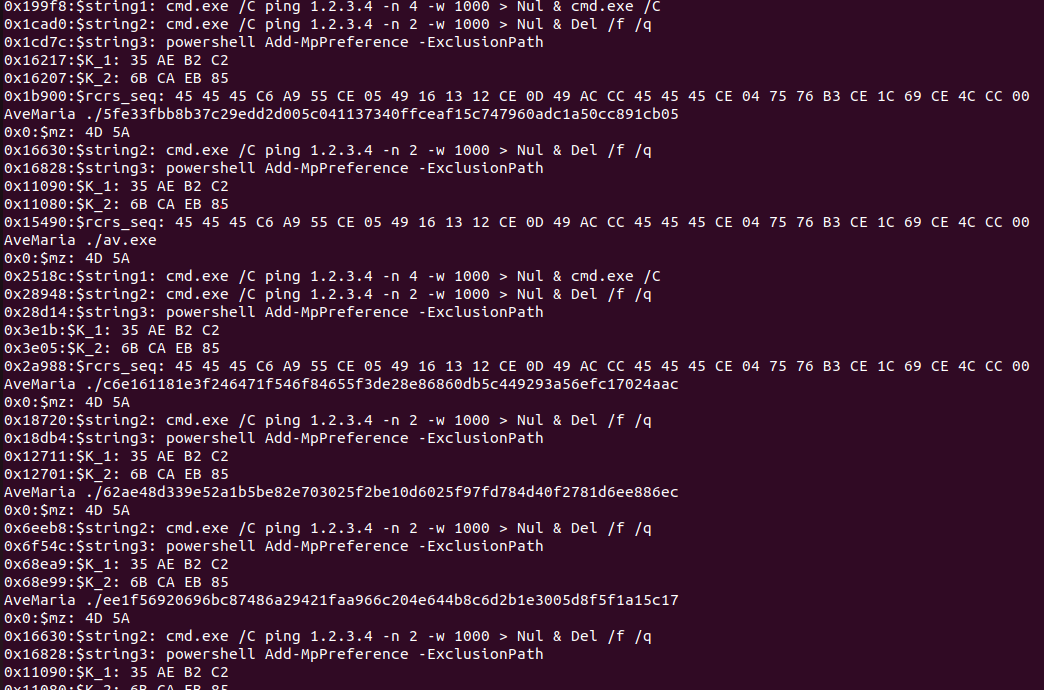we can see that

• string2
• string3
• K_1
• K_2

Found in all of them and the

• rcrs_seq

Found in four of them, and actually, we managed to reach our first objective by detecting all of them with this rule.

# Configuration Extraction

The only important part in the configuration for this family is the C2 server so we need to write a script that extracts them automatically for us.

Starting by looking at where is the address stored and how it’s stored (encrypted or not), we can find that by tracing back the address passed to Internet connection APIs. Or need to perform Some code analysis to find out where is this Configuration stored and how It’s decrypted, after some looking at the code I found that the Configuration for our sample is stored in the `.bss` section and decrypted using `RC4`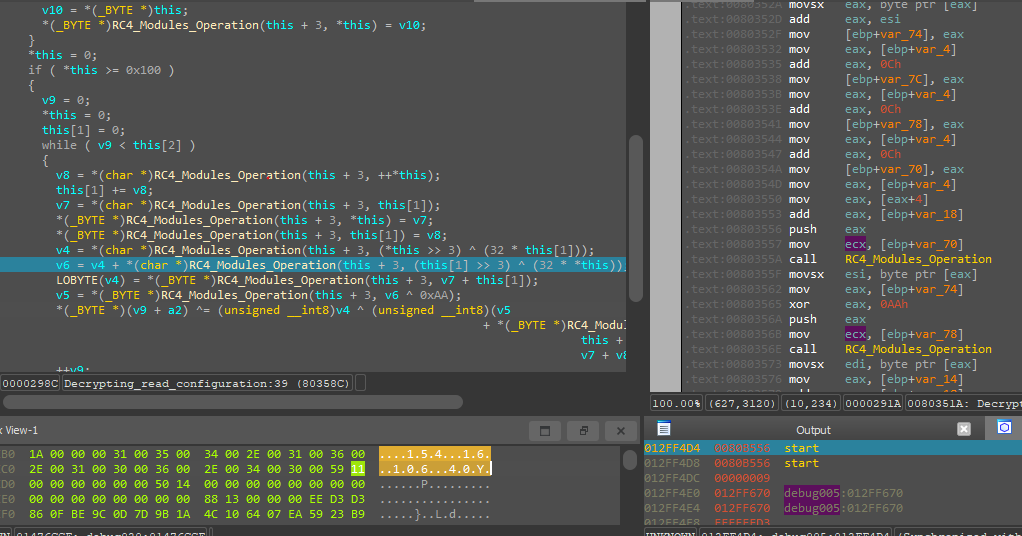Here is the data from the “.bss” section.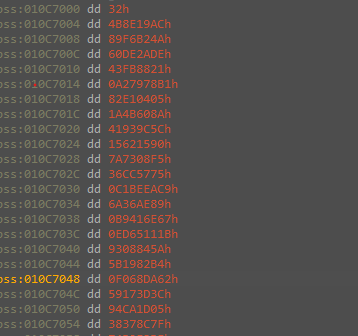And from code analysis we know that the first 4 bytes are the length of the key and the next 0x32h bytes are the key and the rest are the encrypted data.

At the start, I decided to test it using `CyberChef` before beginning to write the python script that we will use as a configuration extractor, but actually it `didn't work`.

After that, I started some debugging and comparison between the code in the sample and the code for the RC4 in Wikipedia and I found the malware uses `nonstandard RC4 implementation`, So we need to understand each part of the code our selves.

Actually, I am not that crypto nerd, I just started looking for the difference between the malware implementation and the standard one, and developing a custom decryptor for it.

That may be easy to say but in practice, it’s painful to implement if you don’t have a deep understanding of the algorithm that you are working with, So I decided to take a different approach and Implement the assembly instruction Sequence that the malware executes to decrypt its configuration inside my python script.

``````Note:
If you have a better approach I hope you can DM me with it.
``````

After some looking at the documentation I was able to clean the decompiled code and define the structure for the rc4 decryption process, and this is the code.

``````void __thiscall rc4(struct_this *this, int data)
{
struct_this *s_box_; // ebx
unsigned int i; // eax
unsigned int index; // eax
int s_box; // edi
int j; // ecx
int v7; // edx
int k; // ecx
char var_k1; // bl
char var_temp; // al
int s_box_1; // edi
char var_k; // [esp+8h] [ebp-10h]
unsigned int cypher; // [esp+10h] [ebp-8h]

s_box_ = this;
cypher = 0;
if ( this->s_box )
{
if ( this->key )
{
this->y = 0;
LOBYTE(i) = 0;
this->x = 0;
do
{
*(_BYTE *)((unsigned __int8)i + this->s_box) = this->x;
i = this->x + 1;
this->x = i;
}
while ( i < 0x100 );
this->x = 0;
for ( index = 0; index < 0x100; this->x = index )
{
s_box = this->s_box;
this->y += *(char *)((unsigned __int8)index + s_box) + *(char *)(index % 0xFA + this->key);
*(_BYTE *)((unsigned __int8)index + s_box) ^= *(_BYTE *)((unsigned __int8)this->y + s_box);
*(_BYTE *)(LOBYTE(this->y) + this->s_box) ^= *(_BYTE *)(LOBYTE(this->x) + this->s_box);
*(_BYTE *)(LOBYTE(this->x) + this->s_box) ^= *(_BYTE *)(LOBYTE(this->y) + this->s_box);
index = this->x + 1;
}
this->x = 0;
this->y = 0;
if ( this->data_len )
{
j = 0;
do
{
s_box_->x = j + 1;
v7 = s_box_->s_box;
k = (unsigned __int8)(j + 1);
var_k1 = *(_BYTE *)(k + v7);
this->y += var_k1;
var_k = var_k1;
var_temp = *(_BYTE *)((unsigned __int8)this->y + v7);
*(_BYTE *)(k + v7) = var_temp;
*(_BYTE *)(LOBYTE(this->y) + this->s_box) = var_k1;
s_box_ = this;
s_box_1 = this->s_box;
*(_BYTE *)(cypher + data) ^= *(_BYTE *)((unsigned __int8)(this->y + var_temp) + s_box_1) ^ (unsigned __int8)(*(_BYTE *)((unsigned __int8)(var_temp + var_k) + s_box_1) + *(_BYTE *)(((unsigned __int8)(*(_BYTE *)((unsigned __int8)((32 * this->y) ^ (this->x >> 3)) + s_box_1) + *(_BYTE *)((unsigned __int8)((32 * this->x) ^ (this->y >> 3)) + s_box_1)) ^ 0xAA) + s_box_1));
j = ++this->x;
++cypher;
}
while ( cypher < this->data_len );
}
}
}
}
``````

The `KSA` and `PRGA` are standard and the decryption loop itself using python is the following

``````while(True):
var_1 = (j+1) % 256
x = var_1
k = (var_1 % 256)
var_k1 = (S[k] % 256)
y = (y + SIGNEXT(var_k1,8))
var_k = SIGNEXT(var_k1,8)
S[k] = S[y % 256] % 256
k = (y %256)
var_temp = SIGNEXT((S[y % 256] % 256),8) % 256
S[k] = var_k1 % 256
k = y
var_2 = (x << 5)
var_3 = (k >> 3)
var_4 = (x >> 3)
F = ((var_2^var_3)%256)
t_3 = SIGNEXT((S[F] % 256),8)
var_5 = (y << 5)
var_6 = (var_4 ^ var_5)
var_7 = var_temp
t_1 = SIGNEXT(S[var_6 % 256],8) % 256
t_2 = t_3 + t_1
t_4 = var_k
N = 0xFFFFFFAA
t_5 = (t_2 ^ N)
t_6= (t_4+var_7)
t_7 = (t_5 % 256)
t_8 = (t_6% 256)
t_9 = ((S[t_8] + S[t_7]) % 256)
t_10 = (y + var_7)
t_11 = (t_9 ^ S[t_10 % 256] % 256) %256
decrypted.append((data[cypher] ^ t_11) % 256)
x = x+1
j = x
cypher = cypher + 1
if (cypher >= len(data)):
break
``````

And I was able to extract the configuration from our samples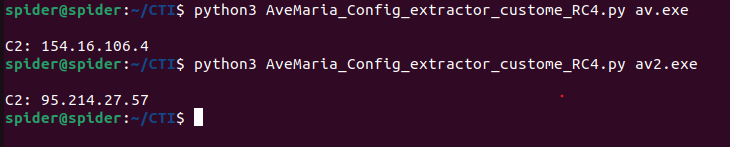Some AveMaria samples come with configuration encrypted using this custom encryption and others are encrypted using the custom implementation of it.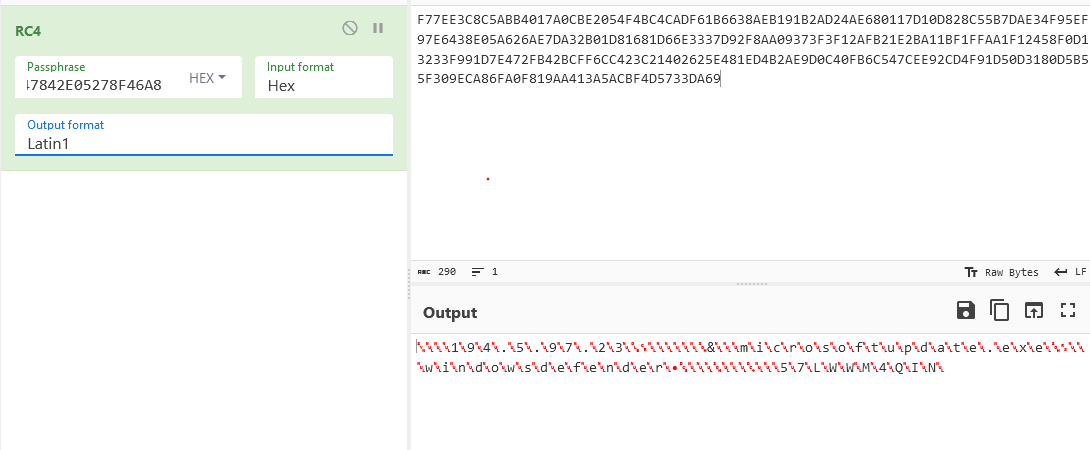Here is a Configuration extractor for those also

``````import pefile
import sys

def ksa(key):
S = list(range(256))
j = 0
for i in range(256):
j = (j + S[i] + key[i % len(key)]) % 256
S[i], S[j] = S[j], S[i]
return S

def prga(S):
i = 0
j = 0
while True:
i = (i + 1) % 256
j = (j + S[i]) % 256
S[i], S[j] = S[j], S[i]
K = S[(S[i] + S[j]) % 256]
yield K

def rc4_decrypt(ciphertext, key):
S = ksa(key)
keystream = prga(S)
plaintext = bytearray()
for c in ciphertext:
plaintext.append(c ^ next(keystream))
return bytes(plaintext)

if len(sys.argv) != 2:
print(f"Usage: python {sys.argv}.py <filename>")
exit()

pe = pefile.PE(sys.argv)
bss_section = pe.sections[-1]
bss_end = bss_start + bss_section.Misc_VirtualSize

bss_data = pe.get_memory_mapped_image()[bss_start:bss_end]
key_length = 50
key = bss_data[4:key_length+4]

data_offset = 0

data_off = 0x0
if (b'\x00\x00\x00\x00\x00\x00\x00\x00\x00\x00' in bss_data[data_offset:]):

data_off = (bss_data[int(data_offset):]).index(b'\x00\x00\x00\x00\x00\x00\x00\x00')

data = bss_data[key_length+4:140]

decrypted_data = rc4_decrypt(data, key)
try :
print(decrypted_data.decode('utf-16-le'))

except:
print(decrypted_data.decode('latin1'))
``````

this works for our test samples…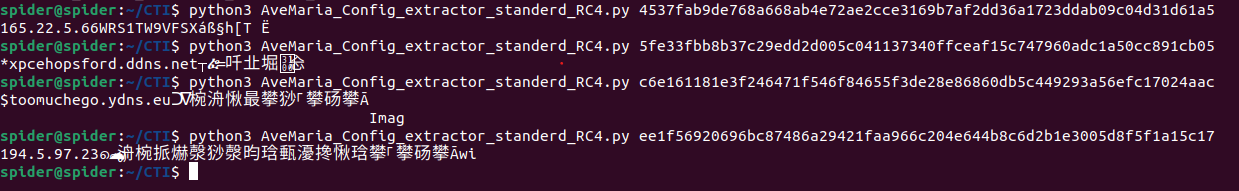``````Note:
During my reasearch, I found AveMaria samples that don't have ".bss" section at all So consider those if they were Caught by the yara rule and the configuration extractor didn't work for them.
``````

Categories:

Updated: